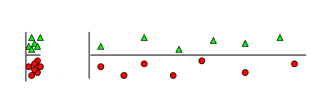Topics: AI | Data Science | Data Visualization | Deep Learning | Machine Learning | NLP | Python | R | Statistics

KDnuggets Home » News » 2014 » Mar » Publications » Machine Learning in 7 Pictures

# Machine Learning in 7 Pictures

Basic machine learning concepts of Bias vs Variance Tradeoff, Avoiding overfitting, Bayesian inference and Occam razor, Feature combination, Non-linear basis functions, and more - explained via pictures.comments
By Deniz Yuret, Feb 2014.

I find myself coming back to the same few pictures when explaining basic machine learning concepts. Below is a list I find most illuminating.1. Bias vs Variance tradeoff - Test and training error: Why lower training error is not always a good thing: ESL Figure 2.11. Test and training error as a function of model complexity.2. Under and overfitting: PRML Figure 1.4. Plots of polynomials having various orders M, shown as red curves, fitted to the data set generated by the green curve.3. Occam's razor ITILA Figure 28.3. This figure gives the basic intuition for why complex models can turn out to be less probable. The horizontal axis represents the space of possible data sets D. Bayes’ theorem rewards models in proportion to how much they predicted the data that occurred. These predictions are quantified by a normalized probability distribution on D. This probability of the data given model Hi, P (D | Hi), is called the evidence for Hi. A simple model H1 makes only a limited range of predictions, shown by P(D|H1); a more powerful model H2, that has, for example, more free parameters than H1, is able to predict a greater variety of data sets. This means, however, that H2 does not predict the data sets in region C1 as strongly as H1. Suppose that equal prior probabilities have been assigned to the two models. Then, if the data set falls in region C1, the less powerful model H1 will be the more probable model.4. Feature combination (1) Why collectively relevant features may look individually irrelevant, and also (2) Why linear methods may fail. From Isabelle Guyon's feature extraction slides.5. Irrelevant features: Why irrelevant features hurt kNN, clustering, and other similarity based methods? The figure above on the left shows two classes well separated on the vertical axis. The figure above on the right adds an irrelevant horizontal axis which destroys the grouping and makes many points nearest neighbors of the opposite class.6. Non-linear basis functions How non-linear basis functions turn a low dimensional classification problem without a linear boundary into a high dimensional problem with a linear boundary.7. Discriminative vs Generative
From SVM tutorial slides by Andrew Moore: a one dimensional non-linear classification problem with input x is turned into a 2-D problem z=(x, x^2) that is linearly separable.

See more Machine Learning pictures at http://www.denizyuret.com/2014/02/machine-learning-in-5-pictures.htmlDeniz Yuret is an associate professor in Computer Engineering at Koç University in Istanbul, Turkey, working at the Artificial Intelligence Laboratory. He was at MIT AI Lab and co-founded Inquira.Get KDnuggets, a leading newsletter on AI, Data Science, and Machine Learning